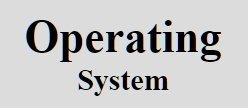# OS Programmed Input/Output

Fundamentally input/output can be performed in one of the following three ways:

In this tutorial, we will learn about the first way to perform the input/output, that is, programmed input/output.

Programmed input/output is the simplest way of input/output.

Simplest way of input/output is to have the central processing unit (CPU) do all the work, that is, programmed input/output, in short.

The figure given below shows the steps to print a story of 6 characters in programmed input/output.The action followed by the OS is programmed input/output is described/summarised in the code given below:

```copy_from_user(buffer, p, count);
for(i=0; i<count; i++)// loop on every character
{
*printer_data_register = p[i];// output one character
}
return_to_user();```

Here, p is the kernel buffer

Note - No doubt, that the programmed input/output is simple but it has a big disadvantage of tying up the CPU full time until all the input/output is done.

Tools
Calculator